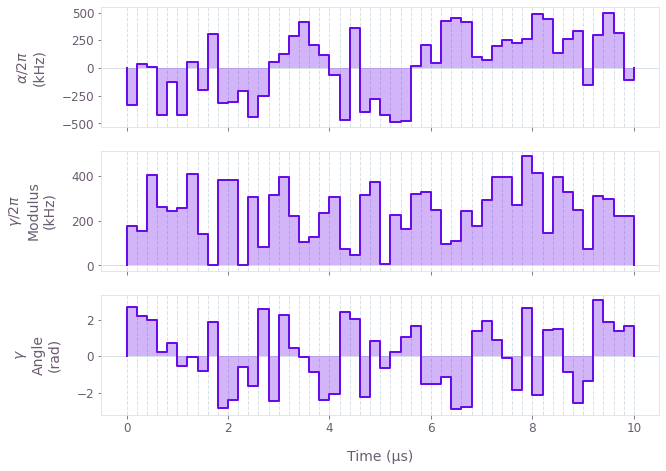# How to optimize controls in D-dimensional systems using graphs

Highly-configurable non-linear optimization framework for quantum control

The Q-CTRL Python package exposes a highly-flexible optimization engine for general-purpose gradient-based optimization. It can be directly applied to model-based control optimization for arbitrary-dimensional quantum systems.

The Q-CTRL python package's optimization engine allows the user to express their system Hamiltonians as almost-arbitrary functions of the controllable parameters. That is, rather than enforcing a specific Hamiltonian structure and allowing the user to define the values of specific operators in that structure, the Q-CTRL optimization engine allows the user to define the entire Hamiltonian. This flexibility is enabled by leveraging the TensorFlow automatic differentiation library. Using simple convenience functions provided by Boulder Opal, the user creates a representation of their system that defines a map from controllable parameters to Hamiltonian. The underlying structure of this map is a TensorFlow graph, which can be efficiently evaluated and differentiated. Once constructed, this mapping (or graph) is then inserted into the optimization cost function calculation. The resulting optimized controls thus achieve the desired objectives, and do so within the constraints imposed by the user-defined Hamiltonian structure. This overall structure makes it trivial to incorporate nonlinearities and constraints on solutions.

## Summary optimization workflow

### 1. Define computational graph

The flexible Q-CTRL optimization engine expresses all optimization problems as data flow graphs, which describe how optimization variables (variables that can be tuned by the optimizer) are transformed into the cost function (the objective that the optimizer attempts to minimize).

Concretely, the qctrl.create_graph function creates a graph which can be fully customized by the user to perform calculations. For an optimization, a typical flow is to:

• Create "signals", or scalar-valued functions of time, which may be non-linear, have enforced temporal structure such as time symmetry, or more generally may depend arbitrarily on the controllable parameters;
• Create "operators", or operator-valued functions of time, by modulating constant operators by signals;
• Combine the operators into a single Hamiltonian operator;
• Calculate the optimization cost function (typically an infidelity from, e.g., an infidelity_pwc graph operation) from the Hamiltonian.

### 2. Execute graph-based optimization

With the graph object created, an optimization can be run using the qctrl.functions.calculate_optimization function. The cost, the outputs, and the graph must be provided. The function returns the results of the optimization. Note this example code block uses naming that should be replaced with the naming used in your graph.

optimization_result = qctrl.functions.calculate_optimization(
graph=graph, cost_node_name="infidelity", output_node_names=["alpha", "gamma"]
)

## Worked example: Robust control of a single qubit

We present a detailed worked example of robust optimization in a single-qubit system. Specifically, we consider a single-qubit system represented by the following Hamiltonian:

\begin{align*} H(t) &= \frac{\nu}{2} \sigma_{z} + \frac{1}{2}\left(\gamma(t)\sigma_{-} + \gamma^*(t)\sigma_{+}\right) + \frac{\alpha(t)}{2} \sigma_{z} + \beta(t) \sigma_{z} \,, \end{align*}

where $\nu$ is the qubit detuning, $\gamma(t)$ and $\alpha(t)$ are, respectively, complex and real time-dependent pulses, and $\beta(t)$ is a small, slowly-varying stochastic dephasing noise process and $\sigma_{\pm}$ are the qubit ladder operators and $\sigma_{z}$ is the Pauli-Z operator.

The functions of time $\gamma(t)$ and $\alpha(t)$ are not predetermined, and instead are optimized by the Q-CTRL optimization engine in order to achieve some target operation.

### Define computational graph

Below we show how to create a data flow graph for optimizing the single-qubit system described above. Comments in the code explain the details of each step.

import matplotlib.pyplot as plt
import numpy as np
from qctrlvisualizer import plot_controls

from qctrl import Qctrl

# Starting a session with the API
qctrl = Qctrl()

# Define standard matrices
sigma_y = np.array([[0, -1j], [1j, 0]])
sigma_z = np.array([[1, 0], [0, -1]])
sigma_m = np.array([[0, 1], [0, 0]])

# Define physical constants
nu = 2 * np.pi * 0.5 * 1e6  # Hz
gamma_max = 2 * np.pi * 0.5e6  # Hz
alpha_max = 2 * np.pi * 0.5e6  # Hz
segment_count = 50
duration = 10e-6  # s

# Define the data flow graph describing the system
graph = qctrl.create_graph()

# Create the time-independent detuning term,
# tensors and arrays are considered constant operators.
detuning = nu * sigma_z / 2

# Create a complex piecewise-constant (PWC) signal, with optimizable modulus
# and phase, representing gamma(t)
gamma = graph.complex_pwc_signal(
moduli=graph.optimization_variable(
count=segment_count, lower_bound=0, upper_bound=gamma_max
),
phases=graph.optimization_variable(
count=segment_count,
lower_bound=0,
upper_bound=2 * np.pi,
is_lower_unbounded=True,
is_upper_unbounded=True,
),
duration=duration,
name="gamma",
)
# Create a PWC operator representing the drive term
drive = graph.pwc_operator_hermitian_part(gamma * sigma_m)

# Create a real PWC signal, with optimizable amplitude, representing
# alpha(t)
alpha = graph.pwc_signal(
values=graph.optimization_variable(
count=segment_count, lower_bound=-alpha_max, upper_bound=alpha_max
),
duration=duration,
name="alpha",
)
# Create a PWC operator representing the clock shift term
shift = alpha * sigma_z / 2

# Create a constant PWC operator representing the dephasing noise
# (note that we scale by 1/duration to ensure consistent units between
# the noise Hamiltonian and the control Hamiltonian)
dephasing = sigma_z / duration

# Create the target operator
target_operator = graph.target(operator=sigma_y)

# Create infidelity
infidelity = graph.infidelity_pwc(
hamiltonian=detuning + drive + shift,
noise_operators=[dephasing],
target=target_operator,
name="infidelity",
)


### Execute graph-based optimization

optimization_result = qctrl.functions.calculate_optimization(
graph=graph, cost_node_name="infidelity", output_node_names=["alpha", "gamma"]
)
print(f"Optimized cost:\t{optimization_result.cost:.3e}")

Your task calculate_optimization (action_id="618012") has started. You can use the qctrl.get_result method to retrieve previous results.
Optimized cost:	3.557e-13


We may use the qctrlvisualizer package to plot the optimized pulses, which are available in the result object.

plot_controls(
plt.figure(),
controls={
"$\\alpha$": optimization_result.output["alpha"],
"$\\gamma$": optimization_result.output["gamma"],
},
)
plt.show()